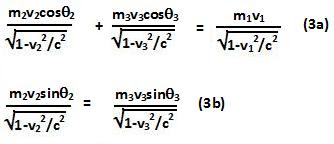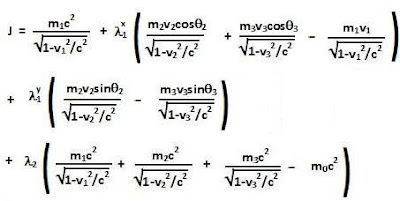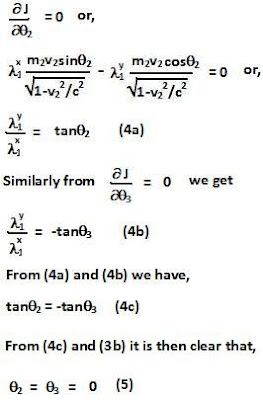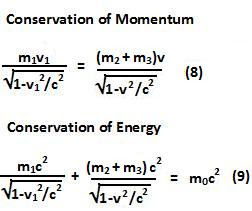## Wednesday, January 13, 2010

### Irodov Problem 1.387Since the mass spontaneously breaks up into three pieces without any external forces both linear mommentum and energy must be conserved. In general, the three
pieces could move along three different directions at velocities v1,v2 and v3
as shown in the figure 1. In that case we have that,From Eqn (1) since the sum of a linear combination of the three velocity vectors is zero (they are linearly dependent) they must be co-planar. This means that without a loss of generality we can restrict the velocity vectors to lie on a plane and choose the direction of velocity v1 to lie along the negative x-axis. This is depicted in figure 2.Now we can simplify (1) as,What choice of velocities and directions will maximize the energy if particle 1? To determine this answer we can use Lagrangian Multipliers to minimize the objective function,The conditions for local extrema are then obtain by setting the partial derivatives of J with respect to each of the unknowns to zero. Hence at extrema we have,Eqn 5 essentially says that in order for the energy of piece 1 to be maximum, both the the pieces 2 and 3 must fly off in the same direction and propel piece 3 in the opposite direction.

Now differentiating with respect to v2 and v3 we have,Eqn 5 and 7 together essentially say that the energy of piece 1 will be maximized when pieces 2 and 3 move at the same velocity and the same direction - in other words they move together. This situation is depcited in Figure 3. Let v2=v3=v.Now equations (1) and (2) can be rewritten as,From (8) we obtain,From (9) we obtain,Eqn (12) given the maximum energy that piece 1 can possible have after the disintegration.